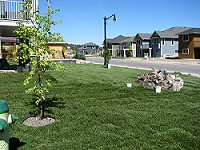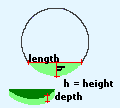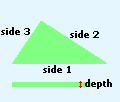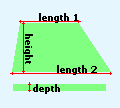Spike's Calculators

# Odd Shapes Turf and Top Soil Calculator - MetricThis calculator will enable you to calculate the square metres of the area you are planning to turf, the amount of topsoil needed in cubic meters and cubic yards, and the amount of turf you need.

Most of the common shapes are included and you can select these as required. It will return values for each of your selections and a total for the combined selections.

If you have an odd shape lawn split it up in sections to match available options as close as possible for the best results.

Since rolls of turf come in different sizes, you will have to calculate the square metres of your roll of turf. Most common roll size covers 1 square metreSquare/RectangleCircleHalf CircleCircle SegmentScalene TriangleTrapazoidRight Angled Triangle

Add the option(s) you require with the following buttons. The total amount of turf and soil needed will be displayed in the box at the bottom of the page.

You will have to remove options you did not use from the form before the final total will be shown.

### The total results will appear here:

 Total Area: M ² Total Topsoil in Cubic Meters: yds ³ Total Topsoil in Cubic Yards: m ³ Total Rolls of Turf: rolls